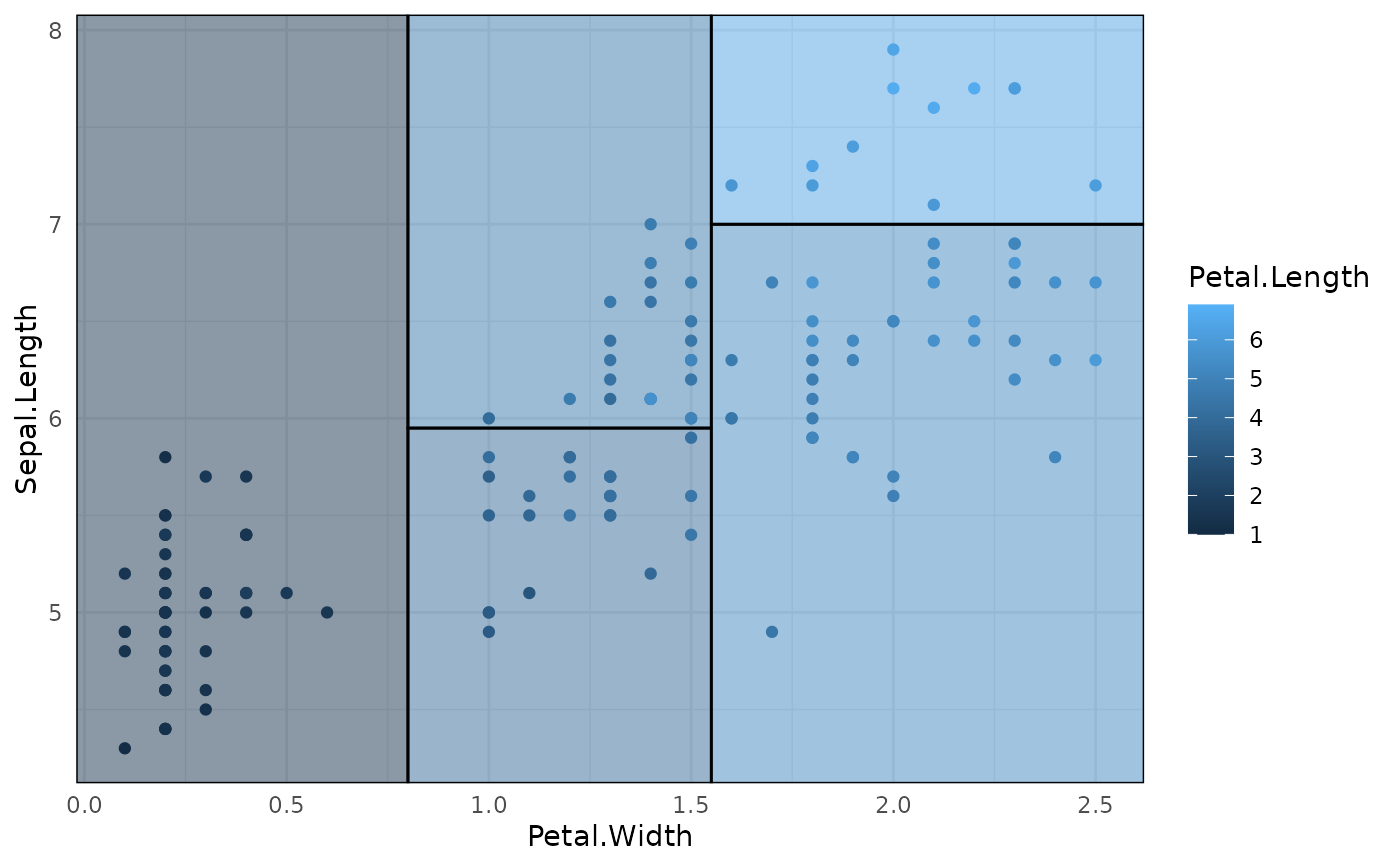geom_parttree() is a simple extension of ggplot2::geom_rect()that first calls parttree() to convert the inputted tree object into an amenable data frame.

## Usage

geom_parttree(
mapping = NULL,
data = NULL,
stat = "identity",
position = "identity",
linejoin = "mitre",
na.rm = FALSE,
show.legend = NA,
inherit.aes = TRUE,
flipaxes = FALSE,
...
)

## Arguments

mapping

Set of aesthetic mappings created by aes(). If specified and inherit.aes = TRUE (the default), it is combined with the default mapping at the top level of the plot. You must supply mapping if there is no plot mapping.

data

An rpart::rpart.object or an object of compatible type (e.g. a decision tree constructed via the partykit, tidymodels, or mlr3 front-ends).

stat

The statistical transformation to use on the data for this layer, either as a ggproto Geom subclass or as a string naming the stat stripped of the stat_ prefix (e.g. "count" rather than "stat_count")

position

Position adjustment, either as a string naming the adjustment (e.g. "jitter" to use position_jitter), or the result of a call to a position adjustment function. Use the latter if you need to change the settings of the adjustment.

linejoin

Line join style (round, mitre, bevel).

na.rm

If FALSE, the default, missing values are removed with a warning. If TRUE, missing values are silently removed.

show.legend

logical. Should this layer be included in the legends? NA, the default, includes if any aesthetics are mapped. FALSE never includes, and TRUE always includes. It can also be a named logical vector to finely select the aesthetics to display.

inherit.aes

If FALSE, overrides the default aesthetics, rather than combining with them. This is most useful for helper functions that define both data and aesthetics and shouldn't inherit behaviour from the default plot specification, e.g. borders().

flipaxes

Logical. By default, the "x" and "y" axes variables for plotting are determined by the first split in the tree. This can cause plot orientation mismatches depending on how users specify the other layers of their plot. Setting to TRUE will flip the "x" and "y" variables for the geom_parttree layer.

...

Other arguments passed on to layer(). These are often aesthetics, used to set an aesthetic to a fixed value, like colour = "red" or size = 3. They may also be parameters to the paired geom/stat.

## Details

Because of the way that ggplot2 validates inputs and assembles plot layers, note that the data input for geom_parttree() (i.e. decision tree object) must assigned in the layer itself; not in the initialising ggplot2::ggplot() call. See Examples.

## Aesthetics

geom_parttree() aims to "work-out-of-the-box" with minimal input from the user's side, apart from specifying the data object. This includes taking care of the data transformation in a way that, generally, produces optimal corner coordinates for each partition (i.e. xmin, xmax, ymin, and ymax). However, it also understands the following aesthetics that users may choose to specify manually:

• fill (particularly encouraged, since this will provide a visual cue regarding the prediction in each partition region)

• colour

• alpha

• linetype

• size

parttree(), ggplot2::geom_rect().

## Examples

library(rpart)

### Simple decision tree (max of two predictor variables)

iris_tree = rpart(Species ~ Petal.Length + Petal.Width, data=iris)

## Plot with original iris data only
p = ggplot(data = iris, aes(x = Petal.Length, y = Petal.Width)) +
geom_point(aes(col = Species))

## Add tree partitions to the plot (borders only)
p + geom_parttree(data = iris_tree)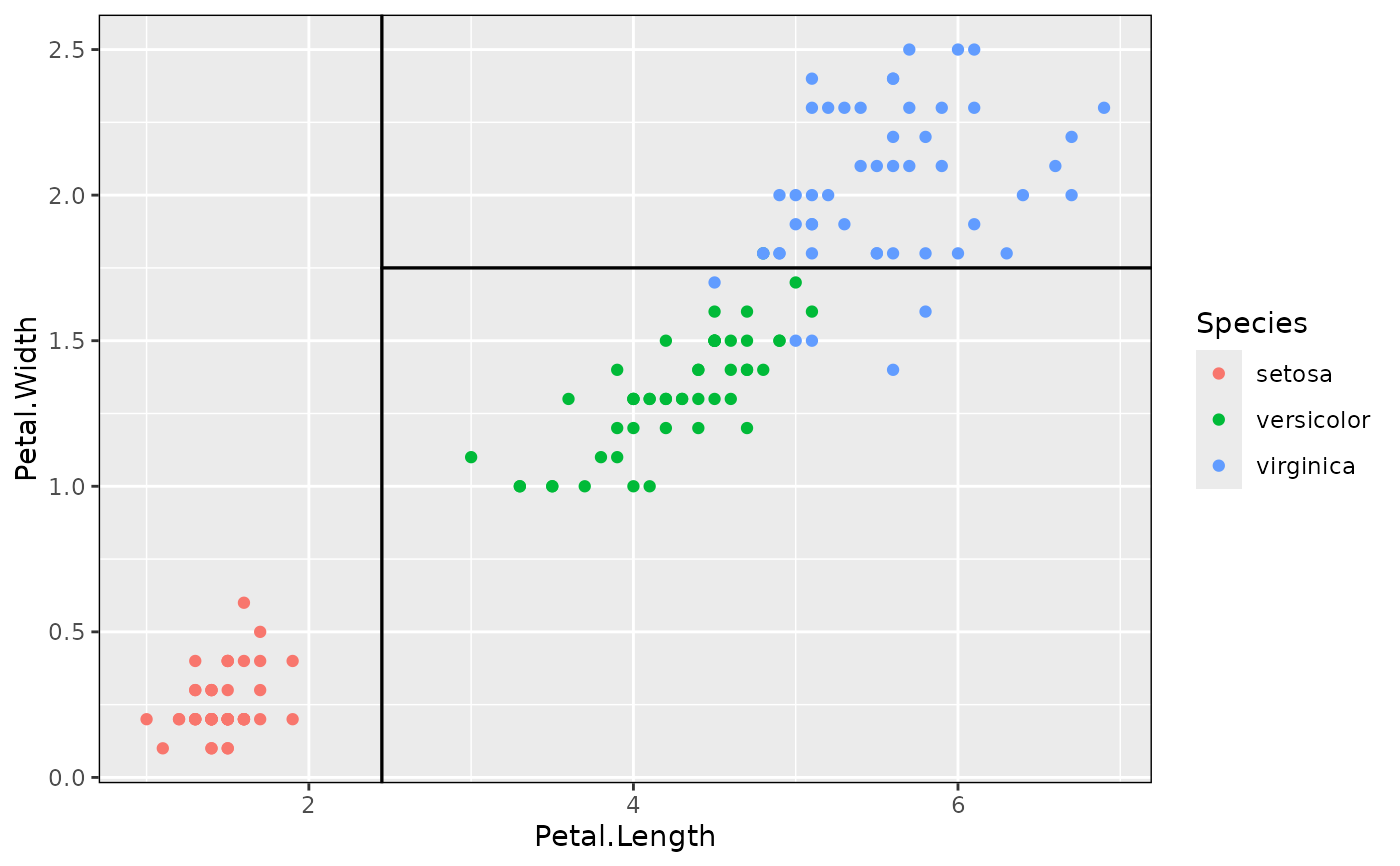## Better to use fill and highlight predictions
p + geom_parttree(data = iris_tree, aes(fill = Species), alpha=0.1)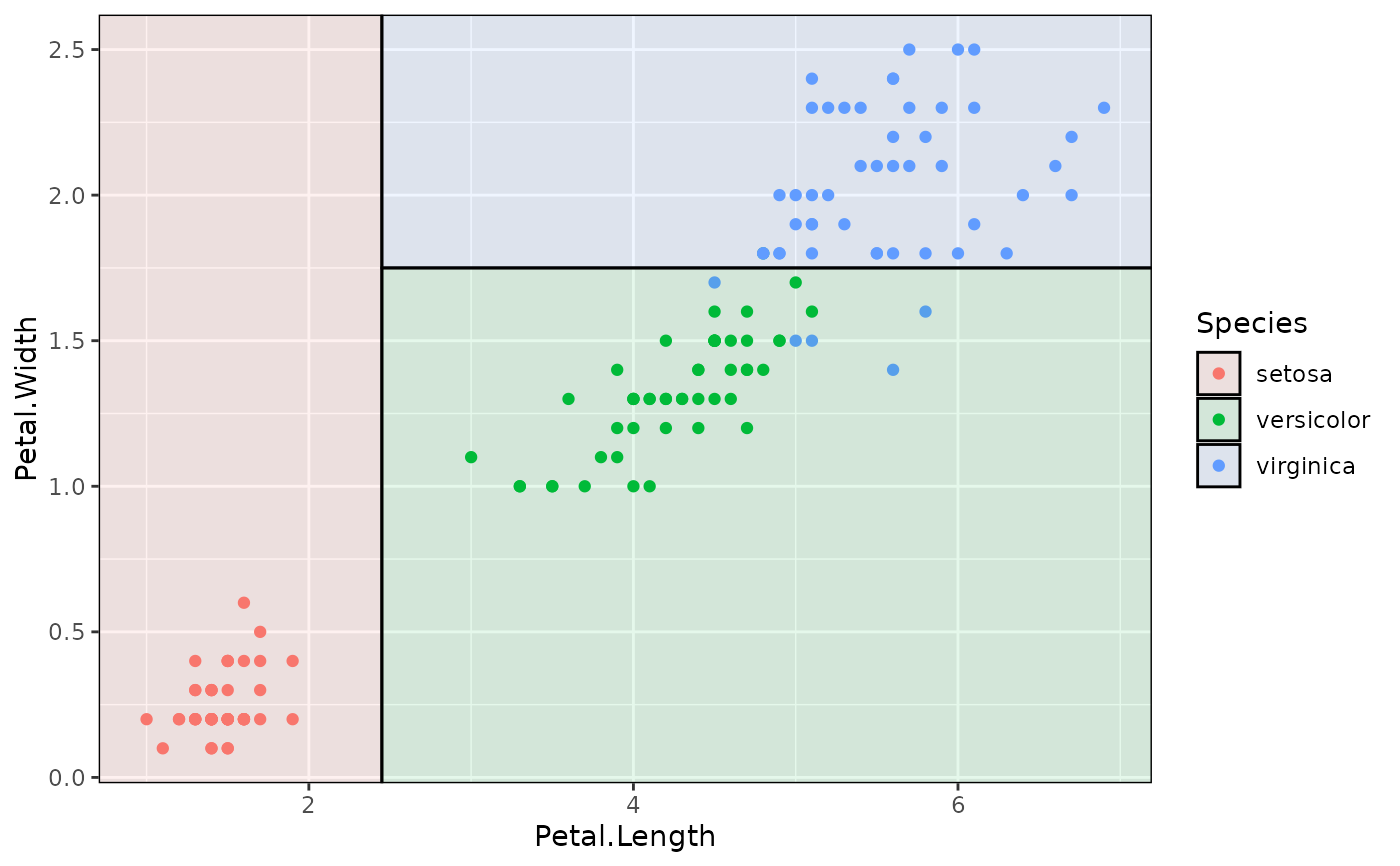## To drop the black border lines (i.e. fill only)
p + geom_parttree(data = iris_tree, aes(fill = Species), col = NA, alpha = 0.1)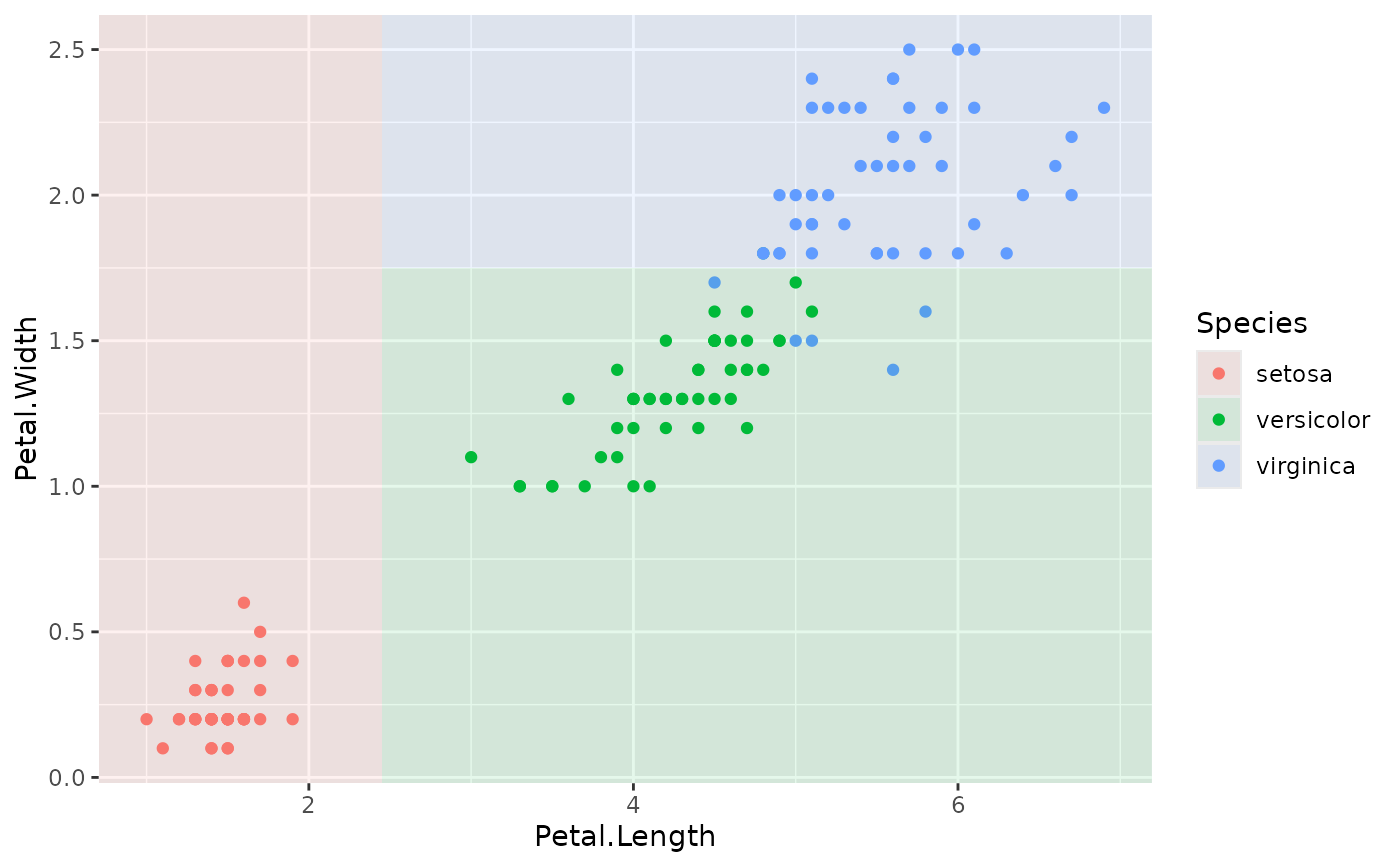### Example with plot orientation mismatch

p2 = ggplot(iris, aes(x=Petal.Width, y=Petal.Length)) +
geom_point(aes(col=Species))

## Oops
p2 + geom_parttree(data = iris_tree, aes(fill=Species), alpha = 0.1)## Fix with 'flipaxes = TRUE'
p2 + geom_parttree(data = iris_tree, aes(fill=Species), alpha = 0.1, flipaxes = TRUE)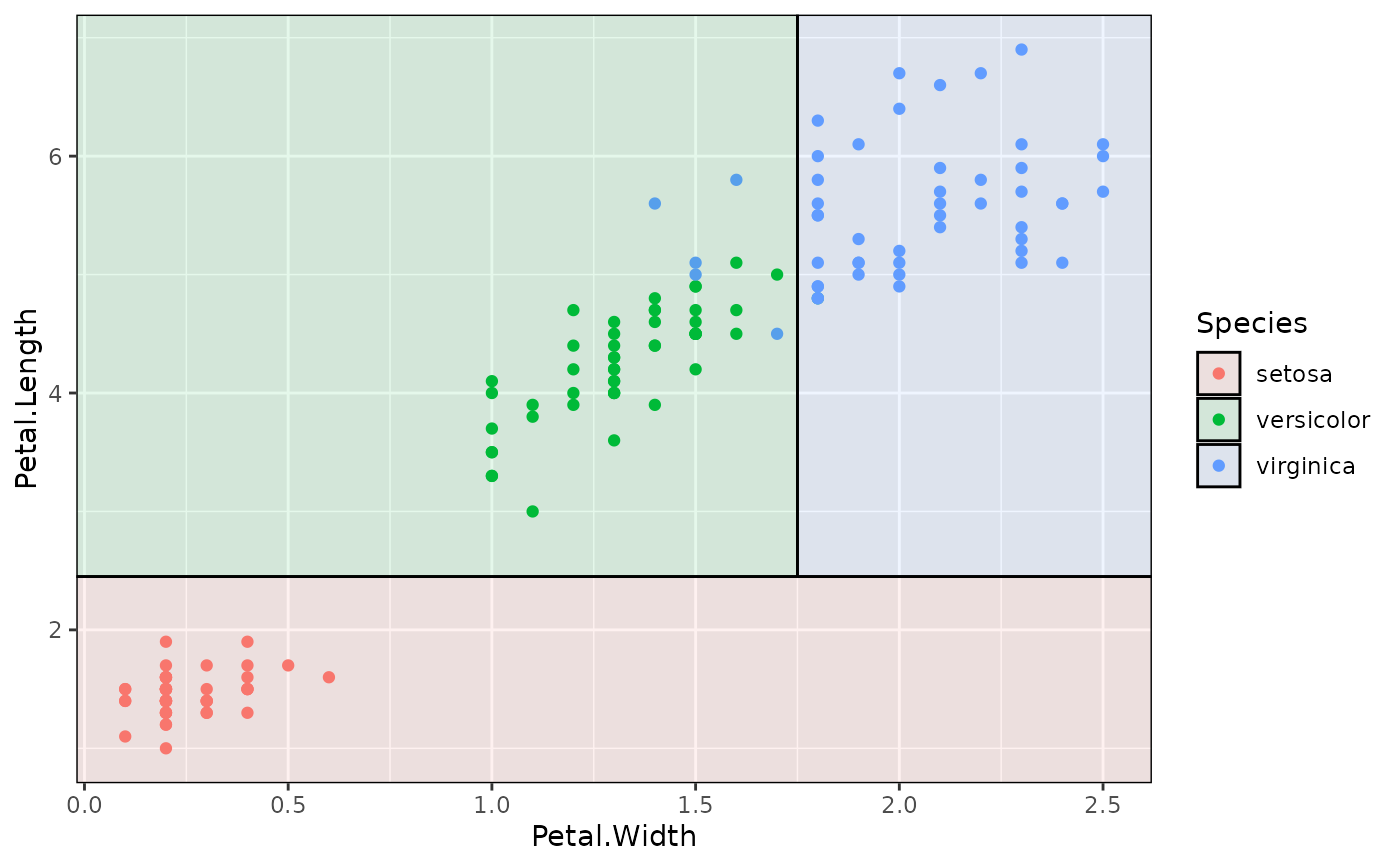### Various front-end frameworks are also supported, e.g.:

library(parsnip)

iris_tree_parsnip =
decision_tree() %>%
set_engine("rpart") %>%
set_mode("classification") %>%
fit(Species ~ Petal.Length + Petal.Width, data=iris)

p + geom_parttree(data = iris_tree_parsnip, aes(fill=Species), alpha = 0.1)### Trees with continuous independent variables are also supported. But you
### may need to adjust (or switch off) the fill legend to match the original
### data, e.g.:

iris_tree_cont = rpart(Petal.Length ~ Sepal.Length + Petal.Width, data=iris)
p3 = ggplot(data = iris, aes(x = Petal.Width, y = Sepal.Length)) +
geom_parttree(
data = iris_tree_cont,
aes(fill = Petal.Length), alpha=0.5
) +
geom_point(aes(col = Petal.Length)) +
theme_minimal()

## Legend scales don't quite match here:
p3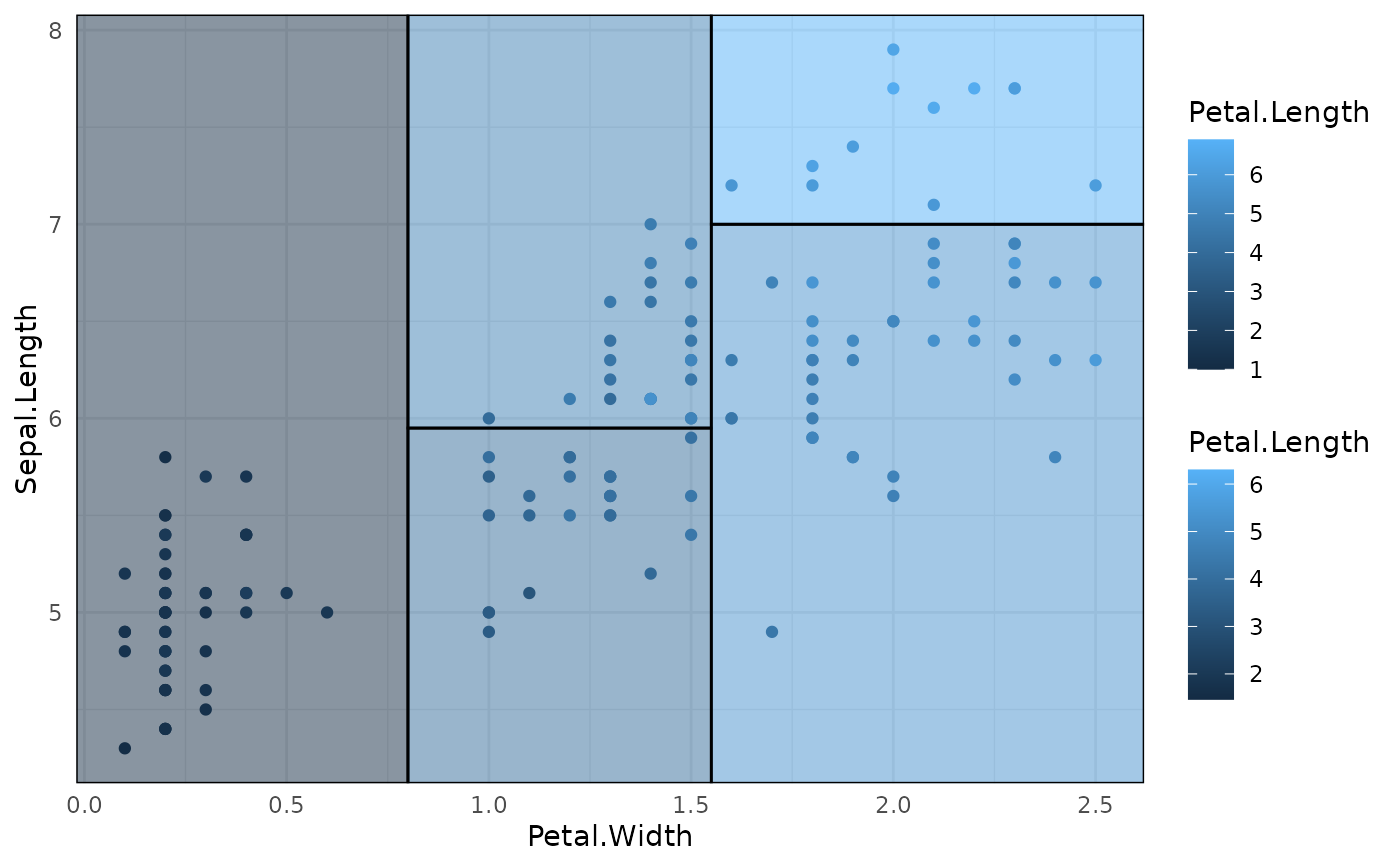## Better to scale fill to the original data
p3 + scale_fill_continuous(limits = range(iris\$Petal.Length))